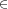## Chapter 15Notes for 10 September

Notes also available as PDF.

### 15.1 Review

#### 15.1.1 Definitions

set
An unordered collection of unique elements.
element
Any item in a set, even other sets. (Also entry, member, item, etc.)
empty set
Or null set. Denoted by rather than \{\}.
singleton
A set with only one element.

#### 15.1.2 Relations

element of
The expression xA states that x is an element of A. If x\mathrel{∉}A, then x is not an element of A.
subset
A ⊂ B if all entries of set A also are in set B.
superset
The reverse of subset. B ⊃ A when A ⊂ B.
proper subset
If all entries of set A also are in set B, but some entries of B are not in A, then A is a proper subset of B.
equality
A = B when A ⊂ B and B ⊂ A.

#### 15.1.3 Operations

union
A ∪ B = \{x\kern 1.66702pt |\kern 1.66702pt xA\text{ or }xB\}.
intersection
A ∩ B = \{x\kern 1.66702pt |\kern 1.66702pt xA\text{ and }xB\}.
set difference
A \ B = \{x\kern 1.66702pt |\kern 1.66702pt xA\text{ and }x∉B\}.
complement and universe
The complement of a set with respect to a given universal set \mathbf{U} is the set {A}^{c} = U \ A = \{x\kern 1.66702pt |\kern 1.66702pt xU\text{ and }x\mathrel{∉}A\}. Sometimes written \overline{A} or A'. Take care with the universal set; there is no “universal” universal set.

### 15.2 From sets to whole numbers

Now we’ll show how to construct whole numbers from sets.

nominal
A number used for indentification, like your student ID.
ordinal
A number used for ordering, as in first, second, etc.
cardinal
A number that counts the number of entries in some set.

We are moving into counting elements of sets. This can extend into probability and other topics, but for now we are interested just in counting and numbers.

Two more definitions are useful:

one-to-one correspondence
Two sets A and B are in one-to-one correspondence if there is a pairing of elements (a,b) such that each aA and bB belongs to exactly one pair. The pairing is also called a bijection.
equivalent sets
Two sets A and B are equivalent if they are in one-to-one correspondence. We write A ~ B for equivalence and A≁B for non-equivalence.

So what do equivalent sets share? Consider establishing a one-to-one correspondence between two sets A and B. If the sets do not have the same number of elements, then at least one element will not be paired. Remember indirect proof, or proof by the contrapositive? We have just proven that all equivalent sets have the same number of elements.

The number of elements is a rather important property and gains its own notation:

cardinality
Writen |A|, or rarely n(A), the cardinality of A is the number of elements in A. So |\{a,b,7\}| = 3.

We now have a link from the cardinality of sets to cardinal integers ≥ 0. These are the whole numbers. Moreover, now we can construct the whole numbers. Starting from the unique empty set, |∅| = 0.

Define an operation S(x) = x ∪\{x\}. This is the successor function. Then S(∅) = ∅∪\{∅\} = \{∅\}, a set with one element. Continue applying the successor to see:

 ∅ |∅| 0 S(∅) |\{∅\}| 1 \{0\} S(S(∅)) |\{∅,\{∅\}\}| 2 \{0,1\} S(S(S(∅))) |\left \{∅,\{∅\},\{∅,\{∅\}\}\right \}| 3 \{0,1,2\}

By applying the successor function n times to the empty set, we obtain a set of cardinality n. Starting from nothing (literally, ), we obtain the whole numbers! This is a construction from Giuseppe Peano, a 19th century Italian mathematician. After the association between and 0, we identify the number k with the set of all numbers preceding k.

The text has examples of building numbers with different physical gizmos. It’s worth thinking about the 2-D block idea, particularly in regards to factors and factorization. Here, you could imagine S as adding one more unit onto a line. Starting from a point, , applying S grows the numbers one at a time.

We also can impose an ordering on these numbers. Given two sets A and B with |A| = a and |B| = b, we say that a < b if A is equivalent to some proper subset of B, or A ~ C where C ⊊ B. We say a ≤ b if A is equivalent to some subset of B.

Now we also can see the difference between finite sets and the concept of infinite sets. A finite set is equivalent to some number of successor applications to the empty set. You can count finite numbers.

An infinite set, however, is equivalent to no number of successor applications. If it were, we could add one more to have a larger set. There are different kinds of infinities, but we won’t worry about that right now. And note that you can have a one-to-one correspondence between infinite sets. For example, \{x\kern 1.66702pt |\kern 1.66702pt x{J}^{+}\} has a one-to-one correspondence with \{{1\over x}\kern 1.66702pt |\kern 1.66702pt x{J}^{+}\}. Each integer in the first set is matched with exactly one in the second set.

### 15.3 Homework

Groups are fine, turn in your own work. Homework is due in or before class on Mondays.

Write out (briefly) your approach to each problem.

• Problem set 2.2 (p97):
• Problems 1, 2, 6, 13, 21, 23